Back to index

ST-TR for the HP-42S Star to Triangle and Triangle to Star conversion

Usage: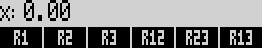Fill-in the known value’s

R1, R2 and R3 when the know value’s are in star, R12, R23, R13 when the know value’s are in triangle.

Press [R/S]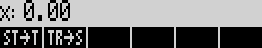Press "ST-> T" for Star to Triangle conversion

Press "TR-> S" for Triangle to Star conversion

Example:

R1=10, R2=20, R3=30

Start to Triangle; Press [ST-> T]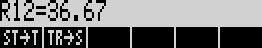Press [R/S]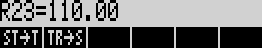Press [R/S]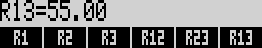And the program is ready for another calculation.

Also you can recall or view the calculated values by pressing [RCL] or [Shift] a menu variable.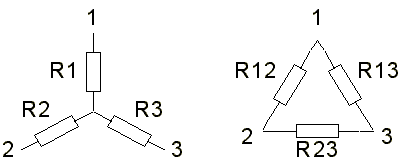Binary files for emulators:
Raw binary: st-tr.raw  Binary for HP-42X: st-tr.42x (HP-48) st-tr49.42x (HP-49)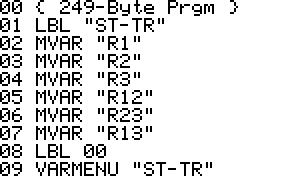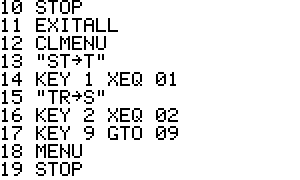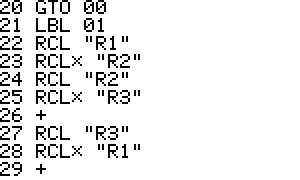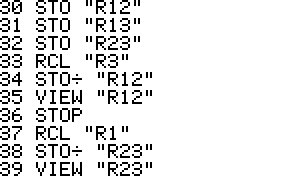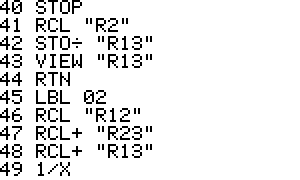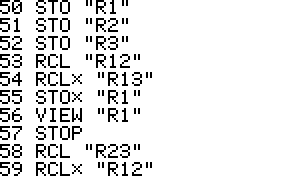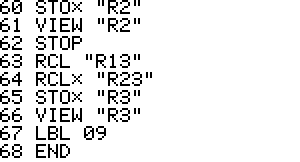Generated by 42s2html - 09 November 2004, 21:30